Next: Gauge invariance Up: Relativity and electromagnetism Previous: The current density 4-vector

## The potential 4-vector

There are many ways of writing the laws of electromagnetism. However, the most obviously Lorentz invariant way is to write them in terms of the vector and scalar potentials (see Sect. 4.6). When written in this fashion, Maxwell's equations reduce to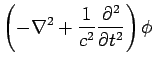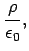(1437)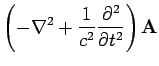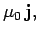(1438)

whereis the scalar potential, and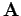the vector potential. Note that the differential operator appearing in these equations is the Lorentz invariant d'Alembertian, defined in Eq. (1409). Thus, the above pair of equations can be rewritten in the form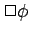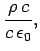(1439)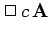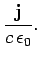(1440)

Maxwell's equations can be written in Lorentz invariant form provided that the entity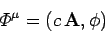(1441)

transforms as a contravariant 4-vector. This entity is known as the potential 4-vector. It follows from Eqs. (1433), (1437), and (1438) that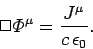(1442)

Thus, the field equations which govern classical electromagnetism can all be summed up in a single 4-vector equation.Next: Gauge invariance Up: Relativity and electromagnetism Previous: The current density 4-vector
Richard Fitzpatrick 2006-02-02